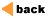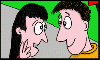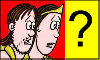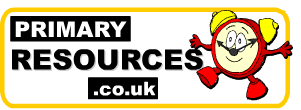Advertisement

Our Other Site: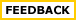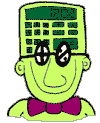Mental and Oral Activities Some quick ideas.... (Some of these activities may be more suitable as an extension or a plenary connected to the main lesson objective.)

 Counting up and down You can count up and down out loud as a whole class, in groups or individually. You could use a number line or a number stick to direct the children. You can count in different steps. You could count down past zero. You should try and vary the starting number and you could set challenges like, "We're going to start at 11 and count up in 3s. What do you think the first number over 30 will be? Multiplying and dividing by ten, a hundred, a thousand Your children will probably soon get quite good at this. You need to teach them beforehand that when you multiply a number by ten the digits move one column to the left (and the opposite for division). I find it useful to write the operation I want the children to do (for example x10) on the board so I can just feed them numbers (individually targetted) to tackle. e.g. 5 to a low ability child (-> 50) and 45.6 to a more able child (->456). I've found that writing up the Th / H / T / U . 1/10th etc. columns on the board provides a support for the less able children. If they're struggling I even write the number to be operated on on the board in the correct columns so that they can visualise how the digits move more easily. Multiplication Strategies My class love doing this simple activity where all you do is put a tricky multiplication fact (e.g. 8x7) in the middle of the board and brainstorm ways that you can get to that fact from other multiplication facts. It's best to present it as "If you didn't know this fact how could you get to the answer." In fact, I've found that instead of asking, "How did you do it?", the children respond better to, "How could you do it?" (for 8x7 you could have many solutions including 4x7 -> double it, 2x7 -> double -> double, 10x7 - 14, 8x8 - 8 or 7x7 + 7 "because we know our square numbers" etc.) Guess the number It's best if you have a number square (on which you can write and rub out) to do this activity. You choose (or a child secretly chooses) a number off the square. The children then ask you questions to identify the number to which you can only answer yes or no. The children may like to cross out the numbers that they eliminate with their questions. After a while your class will probably get very good at getting the answer quickly although they might find it difficult to get past the early temptation to ask "Is it 5?", "Is it 25?" etc. all the time. Introducing a 'life' system helps avoid this. Example questions:- "Is it odd?", "Is it bigger than 10?", "Is it in the five times table?" Doubles and near doubles These are easy enough to practice either by carefully targetted Q&A or by whole class/group responses. Working in examples like 5 pounds plus 5 pounds, 6 minutes plus seven minutes, 4 pounds 50 and 50p etc.help the children towards using their doubles knowledge in other situations. [The same applies to other number facts, e.g. 5 + 3 = 8 helps with 500 + 300] Making numbers Present the children with three digits, e.g. 2, 3 and 7. Can they use these digits to make every number from 0 to (say) 20. e.g. 2 - 2 = 0, 3 - 2 = 1, 3 - 3 + 2 = 2, (3 - 2) x 3 = 3 etc. Discussion comes from the many different ways to create each number. You can change the activity by creating new rules for how the children can use the digits or restricting the number of operations to be used. Number Facts Show two number cards and get the children to give you a number fact using those two cards. E.g. for 3 and 5 you could have 3 + 5 = 8, 5 - 3 = 2, 5 + 3 = 8, 5 x 3 = 15 etc.